# 机器学习必备技能之“统计思维1.0”TUSHARE  金融与技术学习兴趣小组

【工具】Python 3

【数据】tushare.pro 、Datacamp

【注意】本文注重的是方法的讲解，请大家灵活掌握。

01

Seaborn是一个基于matplotlib的Python数据可视化库，能够便于我们作出各种炫酷的统计图形。

① 直方图

import tushare as ts
import pandas as pd
import numpy as np
import matplotlib.pyplot as plt
import seaborn as sns
ts.set_token('your token')
pro = ts.pro_api()
# 取000001的前复权行情
df_close = np.array(df['close'])
print(df_close)
# 设置默认样式
sns.set()
# 画直方图
_ = plt.hist(df_close)
plt.xlabel('000001.SZ_close_price')
plt.ylabel('count')
plt.show()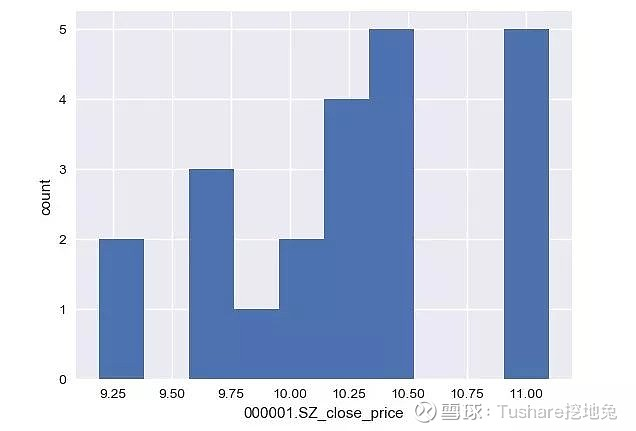# 将bins设置为数据集大小的平方根n_data = len(df_close)
n_bins = np.sqrt(n_data)
n_bins = int(n_bins)
print(n_bins)
# 画直方图
_ = plt.hist(df_close, bins=n_bins)
plt.xlabel('000001.SZ_close_price')
plt.ylabel('count')
plt.show()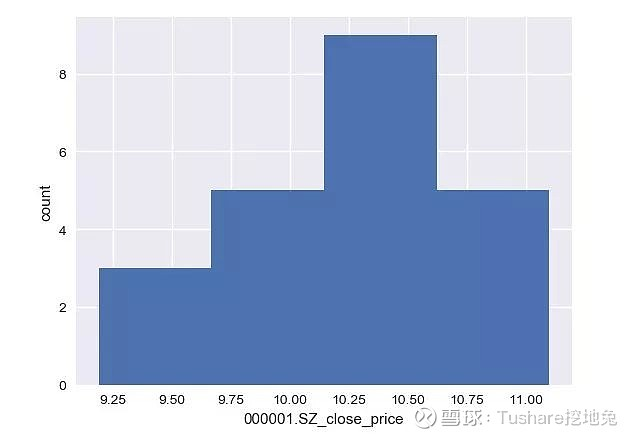② 蜂窝图

# 获取数据：由三只股票合并成的表格code_list = ['000001.SZ', '002142.SZ', '600000.SH']
df_all = pd.DataFrame()
for code in code_list:
print(code)
df_all = df_all.append(df, ignore_index=True)
print(df_all)
# 画图
_ = sns.swarmplot(x='ts_code', y='close', data=df_all)
plt.show()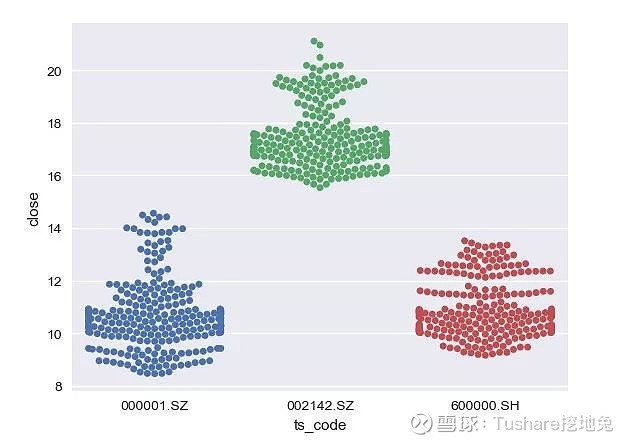③ 经验累积分布函数图ECDF

def ecdf(data):
"""Compute ECDF for a one-dimensional array of measurements."""
n = len(data)
x = np.sort(data)
y = np.arange(1, len(data)+1) / n
return x, y
print(df_close)
x_vers, y_vers = ecdf(df_close)
_ = plt.plot(x_vers, y_vers, marker='.', linestyle='none')
_ = plt.xlabel('000001.SZ_close_price')
_ = plt.ylabel('count')
plt.show()
[ 9.19  9.28  9.75  9.74  9.66  9.94 10.1  10.2  10.11 10.24 10.48 10.25
10.43 10.34 10.28 10.35 10.52 11.   10.94 11.   10.95 11.1 ]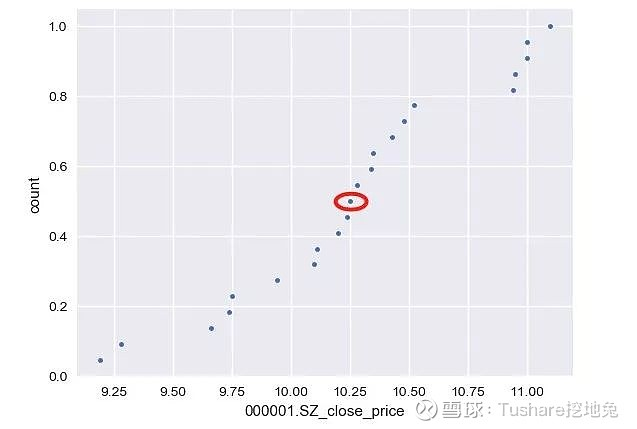02

# 数据集为股票000001.SZ从20190101到20190131的收盘价print(df_close)
[ 9.19  9.28  9.75  9.74  9.66  9.94 10.1  10.2  10.11 10.24 10.48 10.25
10.43 10.34 10.28 10.35 10.52 11.   10.94 11.   10.95 11.1 ]
print('mean:', np.mean(df_close))
print('median:', np.median(df_close))
print('variance:', np.var(df_close))
print('standard deviation:', np.std(df_close))
mean: 10.265909090909089
median: 10.265
variance: 0.2749423553719008
standard deviation: 0.5243494592081704

a = np.array([1, 2, 3, 4, 5])
print(np.percentile(a, 50))
3.0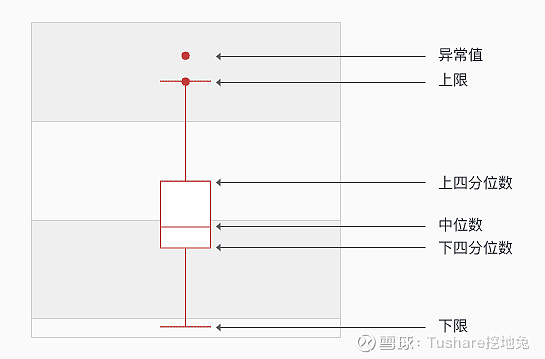Q1的位置 = 1+（n-1）x 0.25

Q3的位置 = 1+（n-1）x 0.75

a = np.array([10, 20, 30, 40])
n = len(a)
Q1_loc = 1 + np.multiply((n-1), 0.25)
Q3_loc = 1 + np.multiply((n-1), 0.75)
print(Q1_loc)
print(Q3_loc)
1.75   # 代表在位置[1，2]区间内，对应的数值区间为[10, 20]
3.25   # 代表在位置[3，4]区间内, 对应的数值区间为[30, 40]
# 计算Q1、Q3
Q1 = np.percentile(a, 25)
Q3 = np.percentile(a, 75)
print(Q1)
print(Q3)
17.5
32.5

Q1 = 0.75 * 20 + 0.25 * 10 = 17.5

Q3 = 0.75 * 30 + 0.25 * 40 = 32.5

Q1和Q3是按照上面的公式计算的，原则就是先确定位置、再根据所在区间计算具体的值。

03

print(np.random.random())
print(np.random.random())
0.8306703392370048
0.7909333011537936

random_numbers = np.random.random(size=100000)
_ = plt.hist(random_numbers)
plt.show()① 0-1分布，也叫伯努利一次试验。

0-1分布是只进行一次事件试验，该事件发生的概率为p，不发生的概率为1-p，比如典型的抛硬币。

② 二项分布，也叫n重伯努利试验。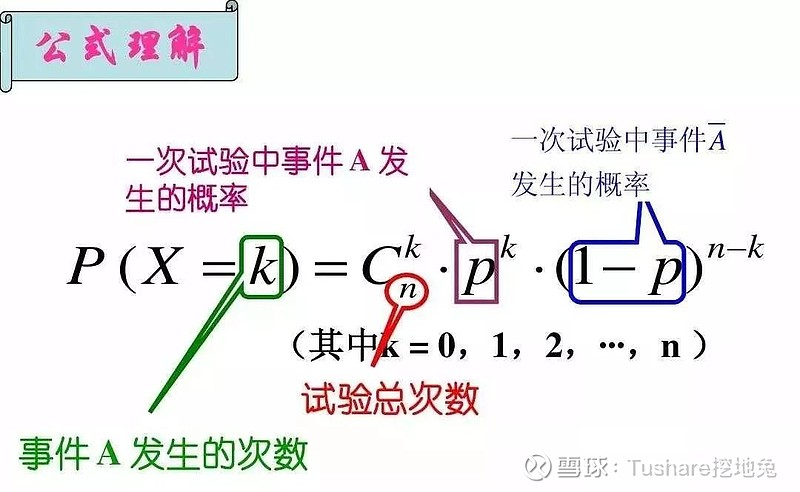s = np.random.binomial(10, 0.5, 100)
print(s)
[5 9 2 2 7 3 4 7 6 5 7 7 5 5 3 6 6 6 5 7 6 7 6 3 7 6 5 6 5 6 3 7 8 7 4 4 4
5 7 3 8 3 2 3 6 2 7 5 6 2 3 5 5 5 7 5 4 7 4 5 3 3 8 4 5 4 4 4 7 3 3 8 7 3
5 4 6 4 3 5 5 6 7 8 2 6 6 6 3 6 7 5 5 3 5 4 6 5 8 4]

p = sum(s == 5) / 100
print(p)
0.26

x, y = ecdf(s)
_ = plt.plot(x, y, marker='.', linestyle='none')
_ = plt.ylabel('CDF')
plt.show()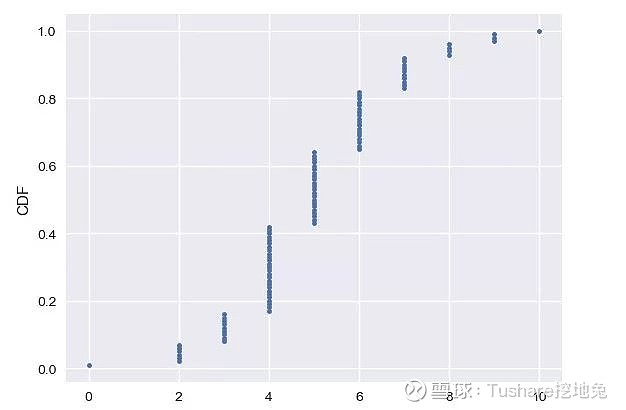③ 泊松分布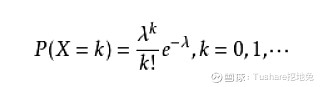s = np.random.poisson(5, 100)
print(s)
[ 3  6  4  7  5  4  7  7  7  5  6  3  0  4  2  2  4  4  5  4  4  5  4  4
4  6 10  2  9  5  5  3  5  5  5  6  7  7  5 10  2  7  7  3  5  4  7  6
1  4 10  4  4  6  6  9  4  9  6  8  6  4  8  7  3  4  3  5  4  3  7  4
5  4  6  4  1  3  1  5  5  5  4  1  7  5  8  1  7  1  4  2  3  4  7  3
8  4  6  3]

# 求每次试验结果大于5的概率p = sum(s > 5) / 100
print(p)
0.35

04

① 正态分布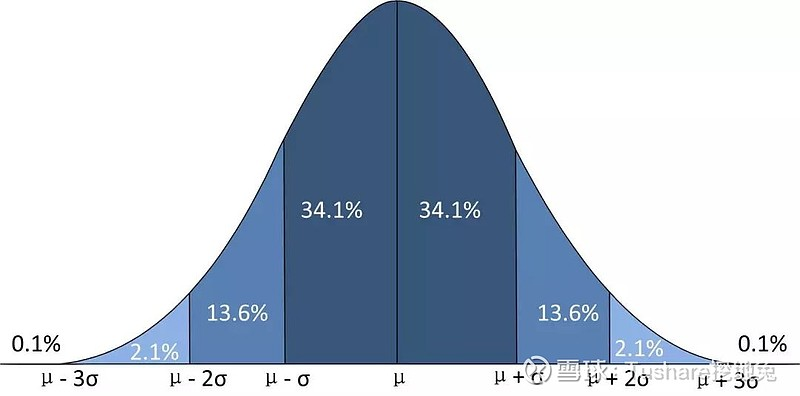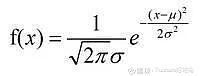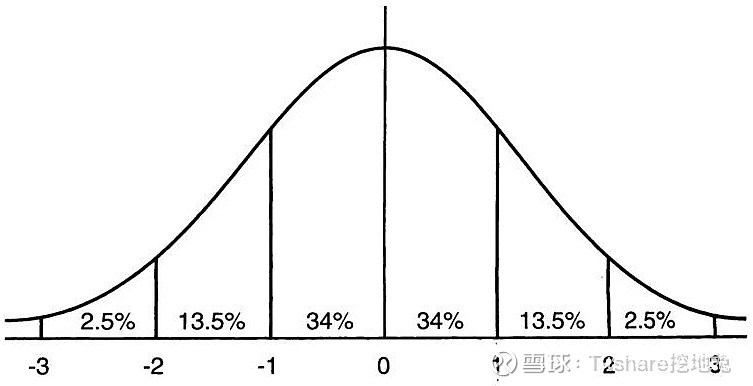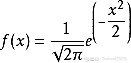samples_std1 = np.random.normal(20, 1, size=100000)
samples_std3 = np.random.normal(20, 3, size=100000)
samples_std10 = np.random.normal(20, 10, size=100000)
# 画图：PDF
_ = plt.hist(samples_std1, bins=100, density=True, histtype='step')
_ = plt.hist(samples_std3, bins=100, density=True, histtype='step')
_ = plt.hist(samples_std10, bins=100, density=True, histtype='step')
_ = plt.legend(('std = 1', 'std = 3', 'std = 10'))
plt.ylim(-0.01, 0.42)
plt.show()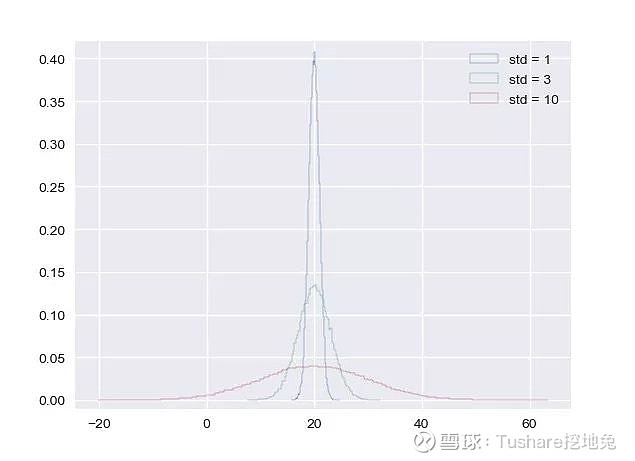# 生成 CDFsx_std1, y_std1 = ecdf(samples_std1)
x_std3, y_std3 = ecdf(samples_std3)
x_std10, y_std10 = ecdf(samples_std10)
# 画图：CDFs
_ = plt.plot(x_std1, y_std1, marker='.', linestyle='none')
_ = plt.plot(x_std3, y_std3, marker='.', linestyle='none')
_ = plt.plot(x_std10, y_std10, marker='.', linestyle='none')
_ = plt.legend(('std = 1', 'std = 3', 'std = 10'), loc='lower right')
plt.show()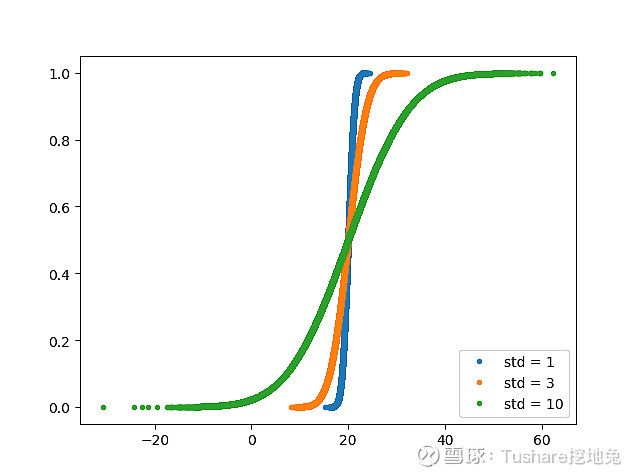samples_mean1 = np.random.normal(0, 1, size=100000)
samples_mean3 = np.random.normal(3, 1, size=100000)
samples_mean10 = np.random.normal(10, 1, size=100000)
# 画图：PDF
_ = plt.hist(samples_mean1, bins=100, density=True, histtype='step')
_ = plt.hist(samples_mean3, bins=100, density=True, histtype='step')
_ = plt.hist(samples_mean10, bins=100, density=True, histtype='step')
_ = plt.legend(('mean = 0', 'mean = 3', 'mean = 10'))
plt.ylim(-0.01, 0.42)
plt.show()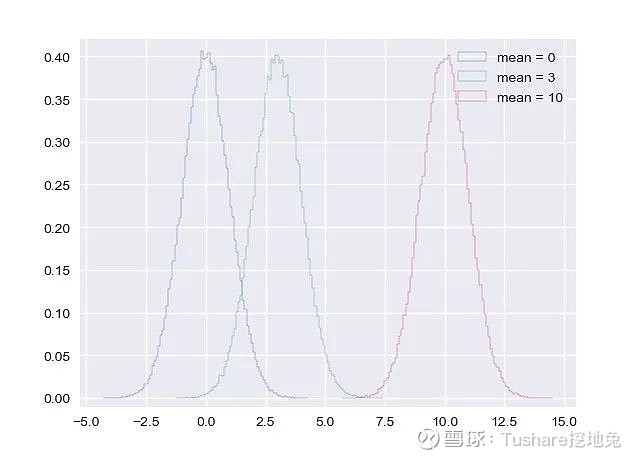# 生成 CDFsx_mean1, y_mean1 = ecdf(samples_mean1)
x_mean3, y_mean3 = ecdf(samples_mean3)
x_mean10, y_mean10 = ecdf(samples_mean10)
# 画图：CDFs
_ = plt.plot(x_mean1, y_mean1, marker='.', linestyle='none')
_ = plt.plot(x_mean3, y_mean3, marker='.', linestyle='none')
_ = plt.plot(x_mean10, y_mean10, marker='.', linestyle='none')
_ = plt.legend(('mean = 1', 'mean = 3', 'mean = 10'), loc='lower right')
plt.show()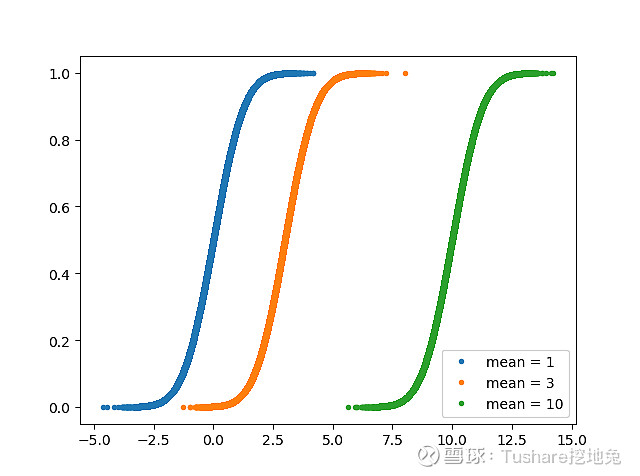samples = np.random.normal(0, 1, size=1000000)
print(samples)
prob = np.sum(samples print('Probability:', prob)
[-1.15137588  2.13435251 -0.23445714 ... -0.41228481 -0.48010039
0.65948965]Probability: 0.840984

② 指数分布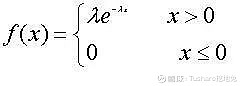P(T>t+s|T>t) = P(T>s)

a = np.random.exponential(300, size=100000)
# 画图：PDF
_ = plt.hist(a, bins=100, density=True, histtype='step')
_ = plt.xlabel('The Exponential distribution')
_ = plt.ylabel('PDF')
plt.show()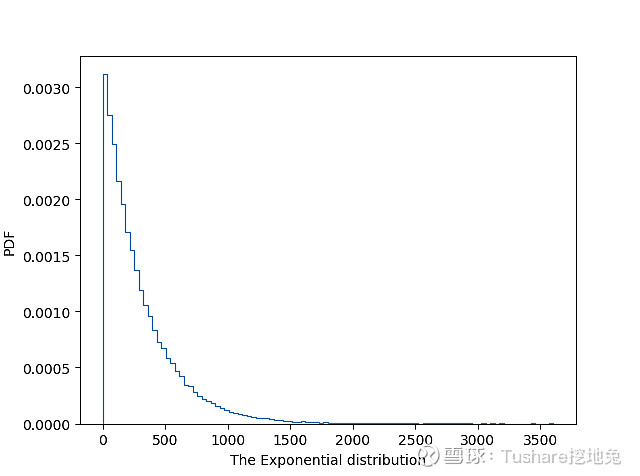# 画图：CDFsx, y = ecdf(a)
_ = plt.plot(x, y, marker='.', linestyle='none')
_ = plt.xlabel('The Exponential distribution')
_ = plt.ylabel('CDF')
plt.show()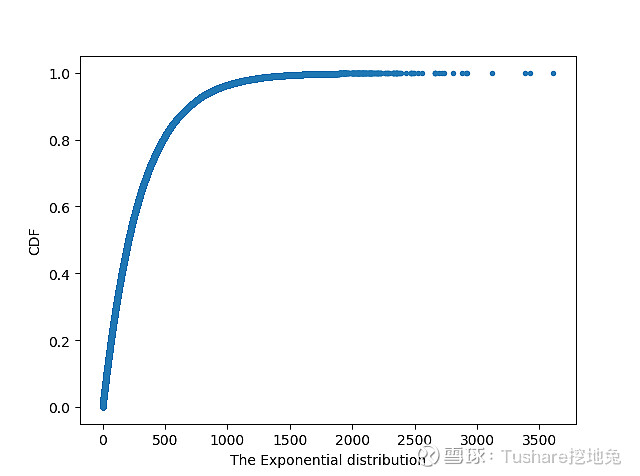05END

【参考链接】

【扩展阅读】机器学习必备技能之“数据预处理”数据科学必备基础之线性回归最简洁的Python时间序列可视化实现Pandas必备技能之“分组聚合操作”Pandas必备技能之“花式拼接表格”

 2019-08-20 10:58 雪球 转发：1 回复：3 喜欢：6

## 全部评论

Tushare挖地兔2019-09-03 11:26